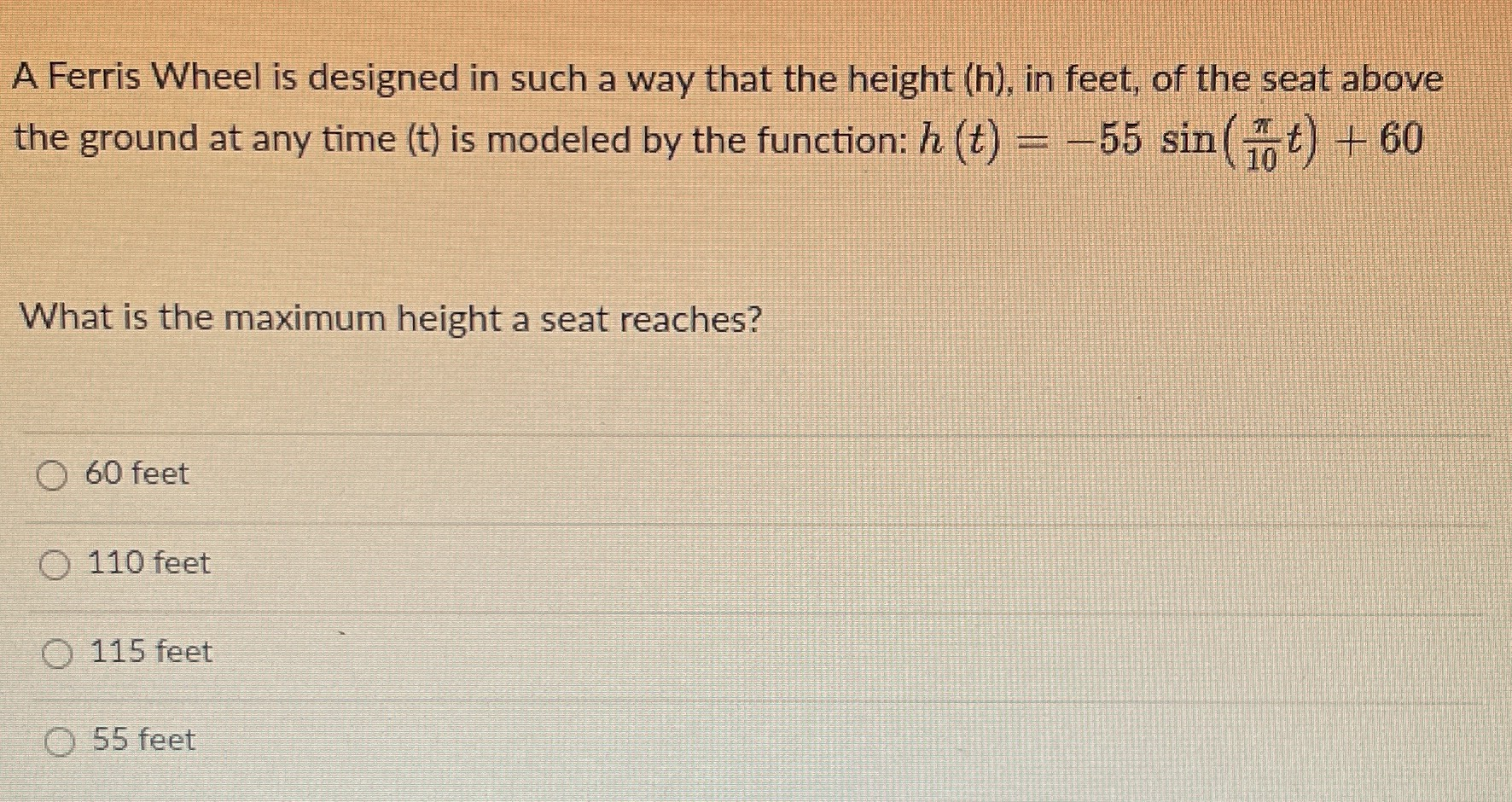### ¿Todavía tienes preguntas de matemáticas?

Pregunte a nuestros tutores expertos
Algebra
PreguntaA Ferris Wheel is designed in such a way that the height $$( h )$$ , in feet, of the seat above the ground at any time $$( t )$$ is modeled by the function: $$h ( t ) = - 55 \sin ( \frac { \pi } { 10 } t ) + 60$$

What is the maximum height a seat reaches?

$$60$$ feet

$$110$$ feet

$$115$$ feet

$$55$$ feet